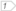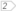Boost C++ Libraries

...one of the most highly regarded and expertly designed C++ library projects in the world.

One minute tutorial

What is a bimap?

A Bimap is a data structure that represents bidirectional relations between elements of two collections. The container is designed to work as two opposed STL maps. A bimap between a collection X and a collection Y can be viewed as a map from X to Y (this view will be called the left map view) or as a map from Y to X (known as the right map view). Additionally, the bimap can also be viewed as a set of relations between X and Y (named the collection of relations view).

The following code creates an empty bimap container:

typedef bimap<X,Y> bm_type;
bm_type bm;

Given this code, the following is the complete description of the resulting bimap. 

• bm.left is signature-compatible with std::map<X,Y>
• bm.right is signature-compatible with std::map<Y,X>
• bm is signature-compatible with std::set< relation<X,Y> >You can see how a bimap container offers three views over the same collection of bidirectional relations.

If we have any generic function that work with maps

template< class MapType >
void print_map(const MapType & m)
{
typedef typename MapType::const_iterator const_iterator;
for( const_iterator iter = m.begin(), iend = m.end(); iter != iend; ++iter )
{
std::cout << iter->first << "-->" << iter->second << std::endl;
}
}

We can use the left map view and the right map view with it

bimap< int, std::string > bm;
...
print_map( bm.left  );
print_map( bm.right );

And the output will be

1 --> one
2 --> two
...
one --> 1
two --> 2
...

Layout of the relation and the pairs of a bimap

The relation class represents two related elements. The two values are named left and right to express the symmetry of this type. The bimap pair classes are signature-compatible with std::pairs.Step by step

A convenience header is available in the boost directory:

#include <boost/bimap.hpp>

Lets define a bidirectional map between integers and strings:

typedef boost::bimap< int, std::string > bm_type;
bm_type bm;

The collection of relations view

Remember that bm alone can be used as a set of relations. We can insert elements or iterate over them using this view.

bm.insert( bm_type::value_type(1, "one" ) );
bm.insert( bm_type::value_type(2, "two" ) );

std::cout << "There are " << bm.size() << "relations" << std::endl;

for( bm_type::const_iterator iter = bm.begin(), iend = bm.end();
iter != iend; ++iter )
{
// iter->left  : data : int
// iter->right : data : std::string

std::cout << iter->left << " <--> " << iter->right << std::endl;
}

The left map view

bm.left works like a std::map< int, std::string >. We use it in the same way we will use a standard map.typedef bm_type::left_map::const_iterator left_const_iterator;

for( left_const_iterator left_iter = bm.left.begin(), iend = bm.left.end();
left_iter != iend; ++left_iter )
{
// left_iter->first  : key  : int
// left_iter->second : data : std::string

std::cout << left_iter->first << " --> " << left_iter->second << std::endl;
}bm_type::left_const_iterator left_iter = bm.left.find(2);
assert( left_iter->second ==  "two" );bm.left.insert( bm_type::left_value_type( 3, "three" ) );

 The type of bm.left is bm_type::left_map and the type of bm.right is bm_type::right_map bm_type::left_-type- can be used as a shortcut for the more verbose bm_type::left_map::-type- This line produces the same effect of bm.insert( bm_type::value_type(3,"three") );

The right map view

bm.right works like a std::map< std::string, int >. It is important to note that the key is the first type and the data is the second one, exactly as with standard maps.

bm_type::right_const_iterator right_iter = bm.right.find("two");

// right_iter->first  : key  : std::string
// right_iter->second : data : int

assert( right_iter->second ==  2 );

assert( bm.right.at("one") == 1 );

bm.right.erase("two");bm.right.insert( bm_type::right_value_type( "four", 4 ) );

 This line produces the same effect of bm.insert( bm_type::value_type(4,"four") );

Differences with std::map

The main difference between bimap views and their standard containers counterparts is that, because of the bidirectional nature of a bimap, the values stored in it can not be modified directly using iterators. For example, when a std::map<X,Y> iterator is dereferenced the return type is std::pair<const X, Y>, so the following code is valid: m.begin()->second = new_value;. However dereferencing a bimap<X,Y>::left_iterator returns a type that is signature-compatible with a std::pair<const X, const Y>

bm.left.find(1)->second = "1"; // Compilation error

If you insert (1,"one") and (1,"1") in a std::map<int,std::string> the second insertion will have no effect. In a bimap<X,Y> both keys have to remain unique. The insertion may fail in other situations too. Lets see an example

bm.clear();

bm.insert( bm_type::value_type( 1, "one" ) );

bm.insert( bm_type::value_type( 1, "1"   ) ); // No effect!
bm.insert( bm_type::value_type( 2, "one" ) ); // No effect!

assert( bm.size() == 1 );

A simple example

Look how you can reuse code that is intend to be used with std::maps, like the print_map function in this example.

#include <string>
#include <iostream>

#include <boost/bimap.hpp>

template< class MapType >
void print_map(const MapType & map,
const std::string & separator,
std::ostream & os )
{
typedef typename MapType::const_iterator const_iterator;

for( const_iterator i = map.begin(), iend = map.end(); i != iend; ++i )
{
os << i->first << separator << i->second << std::endl;
}
}

int main()
{
// Soccer World cup

typedef boost::bimap< std::string, int > results_bimap;
typedef results_bimap::value_type position;

results_bimap results;
results.insert( position("Argentina"    ,1) );
results.insert( position("Spain"        ,2) );
results.insert( position("Germany"      ,3) );
results.insert( position("France"       ,4) );

std::cout << "The number of countries is " << results.size()
<< std::endl;

std::cout << "The winner is " << results.right.at(1)
<< std::endl
<< std::endl;

std::cout << "Countries names ordered by their final position:"
<< std::endl;

// results.right works like a std::map< int, std::string >

print_map( results.right, ") ", std::cout );

std::cout << std::endl
<< "Countries names ordered alphabetically along with"
"their final position:"
<< std::endl;

// results.left works like a std::map< std::string, int >

print_map( results.left, " ends in position ", std::cout );

return 0;
}

The output of this program will be the following:

The number of countries is 4

The winner is Argentina

Countries names ordered by their final position:
1) Argentina
2) Spain
3) Germany
4) France

Countries names ordered alphabetically along with their final position:
Argentina ends in position 1
France ends in position 4
Germany ends in position 3
Spain ends in position 2

Continuing the journey

For information on function signatures, see any standard library documentation or read the reference section of this documentation.

CautionBe aware that a bidirectional map is only signature-compatible with standard containers. Some functions may give different results, such as in the case of inserting a pair into the left map where the second value conflicts with a stored relation in the container. The functions may be slower in a bimap because of the duplicated constraints. It is strongly recommended that you read The full tutorial if you intend to use a bimap in a serious project.

 A type is signature-compatible with other type if it has the same signature for functions and metadata. Preconditions, postconditions and the order of operations need not be the same.